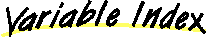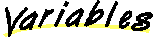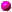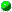# Class FConvexPoly

```java.lang.Object
|
+----FConvexPoly
```

class FConvexPoly
extends Object
The FConvexPoly class represents a convex polygon.

##closedpolyColortrigo
Indicates if the polygon has been drawn in counter-clockwise orderv
The vector used to store the polygon vertices

##FConvexPoly(Color)
Creates a new empty polygon

##addPoint(FPoint)
Takes a point and adds it to the polygon if this point keeps the polygon convex.boundVector()
Returns the vector between the first and last vertices.draw(Graphics)
Draw the outline of the polygon on the given graphics.fill(Graphics)
Draw the filled polygon on the given graphics.firstVector()
Returns the first vector of the polygonal chain.getFPoint(int)
Returns the FPoint indexed by i.getPoint(int)
Returns the Point indexed by i.infiniteRegion(FPoint, FVector, FPoint, FVector)
This method returns a Polygon that will look infinite when drawn by the Graphics methods.isClosed()
Returns true if the polygon is closed.isNextOK(FPoint)
Returns true iff the given point would keep the polygon convex if addded.isTrigo()
Returns true if the polygon has been drawn in counter clockwise order.lastVector()
Returns the last vector of the polygonal chain.

##v
``` public Vector v
```
The vector used to store the polygon verticesclosed
``` boolean closed
```trigo
``` boolean trigo
```
Indicates if the polygon has been drawn in counter-clockwise orderpolyColor
``` Color polyColor
```

##FConvexPoly
``` public FConvexPoly(Color c)
```
Creates a new empty polygon

##isClosed
``` public boolean isClosed()
```
Returns true if the polygon is closed.isTrigo
``` public boolean isTrigo()
```
Returns true if the polygon has been drawn in counter clockwise order.getPoint
``` public Point getPoint(int i)
```
Returns the Point indexed by i.getFPoint
``` public FPoint getFPoint(int i)
```
Returns the FPoint indexed by i. The actual index for these two functions is computed modulo the number of vertices.addPoint
``` public void addPoint(FPoint p)
```
Takes a point and adds it to the polygon if this point keeps the polygon convex. It also updates the "trigo" and "closed" variables when appropriate.isNextOK
``` public boolean isNextOK(FPoint p)
```
Returns true iff the given point would keep the polygon convex if addded. It checks this by making sure the point is on the right side the three lines that define the possible next-point region.firstVector
``` public FVector firstVector()
```
Returns the first vector of the polygonal chain.lastVector
``` public FVector lastVector()
```
Returns the last vector of the polygonal chain.boundVector
``` public FVector boundVector()
```
Returns the vector between the first and last vertices. These three functions return the three vectors that describe the possible next-point regionfill
``` public void fill(Graphics g)
```
Draw the filled polygon on the given graphics.draw
``` public void draw(Graphics g)
```
Draw the outline of the polygon on the given graphics.infiniteRegion
``` public static Polygon infiniteRegion(FPoint p1,
FVector v1,
FPoint p2,
FVector v2)
```
This method returns a Polygon that will look infinite when drawn by the Graphics methods. This should not be in here, it should not be a static method, but it worked so I left it this way...